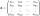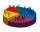Euclidean distance

Calculate the Euclidean distance between shops A, B and C, where:
A 45 0.05
B 60 0.05
C 52 0.09

Wherein the first figure is the weight in grams of bread and second figure is price in USD.

Result

|AB| =  15
|BC| =  8.0001
|AC| =  7.00011

Solution:Leave us a comment of example and its solution (i.e. if it is still somewhat unclear...):Be the first to comment!To solve this example are needed these knowledge from mathematics:

Pythagorean theorem is the base for the right triangle calculator.

Next similar examples:

1. Euclid2In right triangle ABC with right angle at C is given side a=27 and height v=12. Calculate the perimeter of the triangle.
2. Isosceles IVIn an isosceles triangle ABC is |AC| = |BC| = 13 and |AB| = 10. Calculate the radius of the inscribed (r) and described (R) circle.
3. ABS CNCalculate the absolute value of complex number -15-29i.
4. CalculationHow much is sum of square root of six and the square root of 225?
5. SeatsSeats in the sport hall are organized so that each subsequent row has five more seats. First has 10 seats. How many seats are: a) in the eighth row b) in the eighteenth row
6. Sequence 3Write the first 5 members of an arithmetic sequence: a4=-35, a11=-105.
7. Theorem proveWe want to prove the sentence: If the natural number n is divisible by six, then n is divisible by three. From what assumption we started?
8. The determinantThe determinant of the unit matrix equals 7. Check how many rows the A matrix contains.
9. Inverse matrixFind how many times is the larger determinant is the matrix A, which equals 9 as the determinant of its inverse matrix.
10. BallsWe have n identical balls (numbered 1-n) is selected without replacement. Determine 1) The probability that at least one tensile strength number coincides with the number of balls? 2) Determine the mean and variance of the number of balls, which coincides.Given that P = (5, 8) and Q = (6, 9), find the component form and magnitude of vector PQ.In a triangle ABC with the side BC of length 2 cm The middle point of AB. Points L and M split AC side into three equal lines. KLM is isosceles triangle with a right angle at the point K. Determine the lengths of the sides AB, AC triangle ABC.In an arithmetic sequence is given the difference d = -3 and a71 = 455. a) Determine the value of a62 b) Determine the sum of 71 members.Given vector OA(12,16) and vector OB(4,1). Find vector AB and vector |A|.How many different triads can be selected from the group 43 students?Find 75th percentile for 30,42,42,46,46,46,50,50,54The inverse matrix for matrix A has a determinant value of 0.333. What value has a determinant of the matrix A?